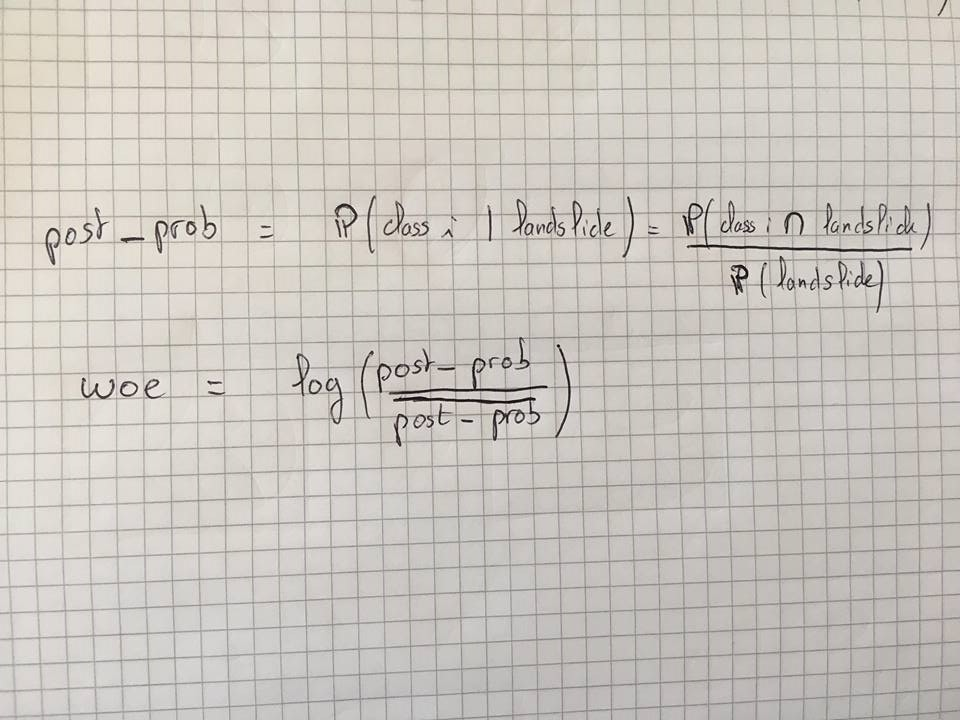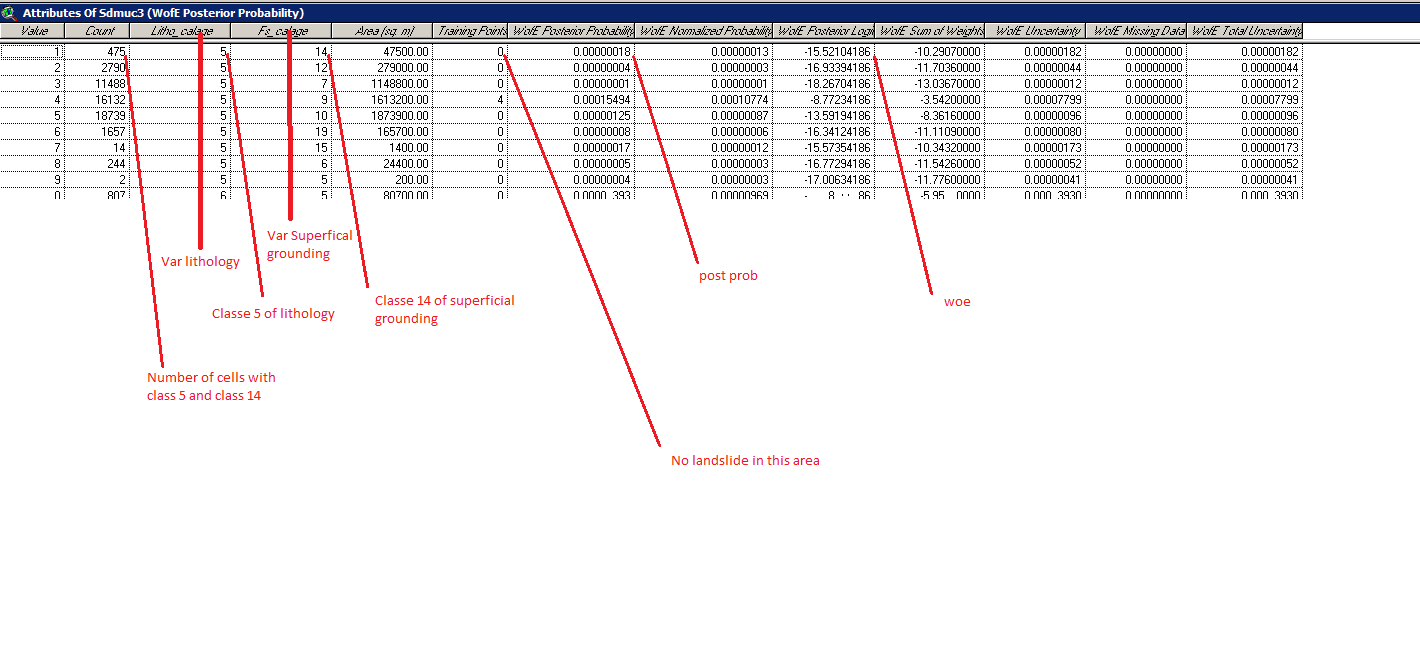# Weight of evidence

3131
17
07-16-2018 05:09 AMNew Contributor III

Hello everybody,

I am working on a project where we are using weight of evidence and i have to implement it on python.

I have some question but not about the implementation, maye i am not in the right place, but if someone knows about woe and want to answer to my question it will be very helpful for me.

Thank you !

Tags (4)
17 RepliesbyMVP Esteemed Contributor

I'm not a statistician and just now learned how to spell WOE, but I found this:

That should just about do it....New Contributor III

Hi Joe,

To set up quickly my project, i am studying the possibilities of landslide on a given area.

In this area we have several variables like lithology or slope, and in each variable we have several classes, for example for slope we can have in degrees 0-5, 5-15, 15-30 ect wich are 3 different classes of slope

In fact if we read the document you sent me or wikipedia or wathever, if i understood correctly we can have a woe (wich means a posterior probability) only if we have a landslide.

we haveso if (class i inter landslide) = 0 we have post prob = 0 and by definition woe = 0

however :here there is no landslide but we have a post prob ..

Do you have any idea why ?New Contributor II

Hello Paul,

I would appreciate if you could provide me me WofE extension for ArcView,  i have been looking for it for a while. my email (elfengourabdo@gmail.com). Thank you in advance.byMVP Legendary Contributor

Showing the code and your reference would be useful if you are wanting to check to see whether your implementation is correct and/or optimized.New Contributor III

In fact this is not a problem with the implementation but maybe a problem of comprehension.

I dont understand why the posterior probability isnt equal to zero whereas there is not landslide in this area.

Here is my code, in fact i want to get the same array i sent a post ago

import numpy as np
import rasterio
#import math
import csv
import os
from itertools import product

def raster_to_numpy(fileraster):
with rasterio.open(fileraster) as src:
if src.meta['nodata'] == None:
print("Warnings : Attention, valeur nodata non renseignée dans le fichier entrée")
nodata = np.array(array.fill_value, dtype=array.dtype)
array = array.filled()
else:
meta = src.meta
meta = meta.values()
nodata = meta[-1]
return array,nodata

def variable(raster,nodata):
variable = list(np.unique(raster))
if (nodata in variable):
variable = np.delete(variable,variable.index(nodata))
return variable

FileDossierRaster = r"C:\Users\user\Desktop\Boulot\Dossier charge"
fileglissement = r"C:\Users\user\Desktop\Boulot\landslides_tests\ASCII\scarp_rb.asc"
fileout = r"C:\Users\user\Desktop\Boulot\Fichiers_csv\sdmuc1.csv"

def attributes(FileDossierRaster,fileglissement,fileout):
liste_raster = []
liste_nodata = []
liste_var = []

for i in os.listdir(FileDossierRaster):
if not os.path.isdir(i):
new_path = os.path.join(FileDossierRaster,i)
raster_var,nodata = raster_to_numpy(new_path)
liste_raster.append(raster_var)
liste_nodata.append(nodata)

v_ras = variable(raster_var,nodata)
liste_var.append(v_ras)

raster_gli,nodata_gli = raster_to_numpy(fileglissement)

taille = [len(i) for i in liste_var]
n = np.prod(taille)

valeurs = np.zeros((n,11+len(liste_raster)))
combi = list(product(*liste_var)) ## ici je fais les combinaisons

for i in range(n):
valeurs[i,0] = i+1
valeurs[i,1:len(combi)+1] = combi ## ici je les mets dans mon tableau
t = 1
for k in range(len(liste_raster)):
t *= np.where(liste_raster == valeurs[i,k+1],1,0) ## possibilé erreur au dernier
c = np.count_nonzero(t == 1)
g = np.where(raster_gli == 1,1,0)
s = np.count_nonzero(t*g == 1)
valeurs[i,len(combi)+1] = c
valeurs[i,len(combi)+2] = s

###########writing csv file#################
entete = ['numero']
for i in range(len(liste_var)):
v = 'variable' + str(i)
entete.append(v)

entete = entete + ['intersection','Cellules_affectees','Proba_posteriori','c','d','e','f','g']
with open(fileout,'w') as f:
w = csv.writer(f, delimiter = ';')
w.writerow(entete)
for line in          zip(valeurs[:,0],valeurs[:,1],valeurs[:,2],valeurs[:,3],valeurs[:,4],valeurs[:,5],valeurs[:,6],valeurs[:,7]):
w.writerow(line)
#######################################
attributes(FileDossierRaster,fileglissement,fileout)byMVP Legendary Contributor

Paul... your column WOE  10**(-15.ish) is about zero.  Are you expecting actual 0.0?New Contributor II

Hi Everyone,

I'm working on Landslide susceptibility mapping wherein I've planned to use the Weight of evidence. the independent variables that I am using are slope. aspect, LULC, distance from the road, geology, geomorphology, etc I wanted to know what are the tools that I can use for  WofE and how to calculate WofE for the above-mentioned variables. kindly, help me to solve this issue. I deeply appreciate your help and considerationNew Contributor III

Hello,

If you used to work with python i've made a program that Can calcul wofe or a particular variable (landslide for exemple) with different raster as input.

Pm me and i will send you the codeNew Contributor II

His Sir,

Thanks for the response. Yeah, I would like to use landslide

areas as dependent variable and slope, aspect, distance from lineaments,

geology and geomorphology as independent variables. can you send me the

python code it will be very helpful ?

Yours Faithfully,

S. Parthipan,

M.Tech, Geoinformatics,

Department of Geography,